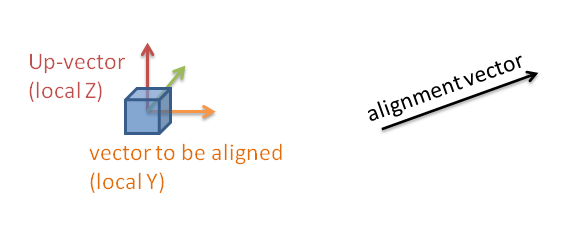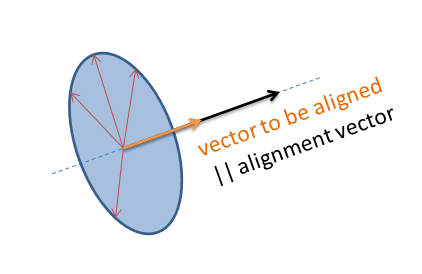# alignAxisToVect Warps Object's Y Rotation of Axis

I am using this:

``````owner.alignAxisToVect(cameraRay.hitPosition, 1, 1)
``````

I am making an object look towards the ray.hitPosition. But the object rotates along the Y axis [especially if you make circles with the ray hit point (CW or CCW)]. I had this happen before with mouse look where the UpDown and LeftRight were on one object. . .

Thanks

Hi cam.dudes,
the problem is in the vector you are using. You are using a position vector instead of direction so the code will work only if the owner is located at coordinates 0,0,0.
To get it to work properly you will have to get the vector from the object to the hit position. I think you can use dir = owner.getVectTo(cameraRay.hitPosition) and then owner.alignAxisToVect(dir, 1, 1).

That makes it do it more. Bleh

Maybe I didn’t describe that well enough. The object starts like this | and when the ray changes positions it looks like this / then this __

Well, the only thing I can think of right now is that you should align one more axis. Try owner.alignAxisToVect((0,0,0),2) after aligning the y axis.

Still does it. The Y axis goes off to the left or right when I move the ray left or right.

if you not need to interpolation(that have alignAxisToVect())

you can use a function of the vector that return a quaternion already “aligned correctly” (the transformation in matrices is automatically done from bge)

obj.worldOrientation = vec.to_track_quat(“Y”, “Z”)

where Y is the axis to align and Z is the axis to keep UP.

anyway Josip is right, not should be the hitPoint the vector to align , i guess it work just by chanceThis is correct behavior. I will explain why:A) your object has 3 local axis (vectors), you defined Y as the vector to be aligned
B) you want to turn the object that the “vector to be aligned” is parallel to the “alignment vector”

First you need to determine the “alignment vector”. As Josip already posted, do not confuse a vector with a point in space. A vector itself has no location. It is a direction and a length. A point has no length or direction. A point in space is usually described as a vector starting from a reference point (usually the origin). Such vectors are called location vectors. So you simply “add” a vector with a given point. As the origin is (0,0,0) the terms of the resulting location vector matches the terms of the vector itself.

In your case you need the vector not the point. When your target point is not in origin you can get the vector by subtracting the location of the object to align to with the location of the object to be aligned (vectors in world space).
Example:

``````
alignmentVector = hitPosition - owner.worldPosition

``````

Btw. the length of the vector does not matter as long as it is non zero (Null vector).

“Aligning” an object along an vector is not unique.The object can have any orientation as long as “vector to be aligned” is parallel to the “alignment vector”. This is exactly what you describe. The image shows you that.

To make this operation unique you need an additional parameter. Usually you define an “up-vector”. The up-vector is an axis of the object that is not parallel to the “vector to be aligned”. To make it easier a common choice is one of the other axis.

As the name “up”-vector indicates this vector should point along global Z.How to do this in code?
This is pretty simply:

1. you align the objects “up-vector” with “global Z”
2. you align the objects “vector to be aligned” to the “alignment vector”
``````
from mathutils import Vector
X = 0
Y = 1
Z = 2
GLOBAL_Z = Vector([0,0,1])

...
alignmentVector = hitPosition - owner.worldPosition
upVector = GLOBAL_Z
owner.alignAxisToVect(upVector, Z)
owner.alignAxisToVect(alignmentVector, Y)

``````

Attention: This solution still has two non-unique situations. This is when the “alignment vector” is parallel to the global Z-Axis.
I guess this can be solved by aligning the remaining local axis (X) along global X or global Y first.

ahem,

so you need
if hitObject != EMPTY:
if hitPos != EMPTY:
Do code

``````
object.alignAxisToVect(hitNormal,1)
object.alignAxisToVect(hitNormal,0)
object.worldPosition = hitOb.worldPosition + hitNormal.normalized() * distance
if object.worldPosition == hitOb.worldPosition + hitNormal.normalized() * distance:
object.setParent(hitOb,0,0)

``````

Check out the “Parent Section” of cube.py

LEft click = pick up block

Right click when holding = Do what you are asking

Note - Made for blender 2.66.1

### Attachments

You guys gave me the help. But I got a local point for the up vector:

``````upVector = owner.getVectTo([owner.localPosition,owner.localPosition, owner.localPosition + 1])
``````

I couldn’t get localOrientation to work

Thanks!

Why you need vecTo()? It is a pretty useless function

• getVectTo returns a result set, not just a vector you are looking for.

You can get the vector just by subtracting the location vectors of both points (point position - object position). Indeed you need vectors first:

``````
target = owner.localPosition.copy()
target.z += 1
print (target - owner.localPosition)

``````

or much easier:

``````
from mathutils import Vector
...
vector = Vector([0,0,1])

``````

To be a little bit more precise to my above explanation, the UP-Vector is not really parallel along global Z. It tries to point along this direction with the smallest possible angle between UP-Vector and global Z.

With the described code the first alignment makes the Up-Vector parallel to global Z and the second alignment applies the requested orientation. While doing this the Up-Vector will turn away from global Z usually with the least change.

. . . Up-Vector parallel to global Z and the second alignment applies the requested orientation.

I did do it you’re described way (maybe I did it wrong) and the result was the object couldn’t rotate along the X axis. Like this --> :no: Okay. . . Nevermind. Local does the same thing.

Josip said in his first post to use getVectTo instead of using a point.If you align the Y axis (ob.alignAxisToVect( Direction, 1), 1 is the Y axis) then you can rotate the object around the Y axis but the objects Y axis will always point in the Direction Vector you defined. You can rotate that object around the Y axis only.

What are you trying to accomplish here ?

A mounted gun. I would imagine setting the Y axis orientation would fix this, but localOrientation is a piece of crap to use.

I do not know what you want to do with localOrientation.

Anyway, if you have a mounted gun, the Up-Vector should try to match the Z-Vector of the parent (rather than global Z). If there is no parent you can use global Z.

Applied to the above code snippet:

``````
...
upVector = calculateUpVector(owner)
...
def calculateUpVector(gameObject):
parent = gameObject.parent
if parent:
return parent.worldOrientation * GLOBAL_Z
else:
return GLOBAL_Z

``````

Let me know if you need a demo blend.

Why couldn’t I set the Y orientation to zero?

I looked at this, and tried setting it like this:

``````owner.localOrientation = 0
owner.localOrientation = 0
owner.localOrientation = 1
``````

It works, except it warps the object itself.